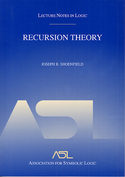# Lecture Notes in Logic 1

## Recursion Theory

Joseph R. ShoenfieldYear: 2001
ISBN: 1-56881-149-7
96 pages. Paperback.

In this volume, the first publication in the Lecture Notes in Logic series, Shoenfield gives a clear and focused introduction to recursion theory. The fundamental concept of recursion makes the idea of computability accessible to a mathematical analysis, thus forming one of the pillars on which modern computer science rests. This introduction is an ideal instrument for teaching and self-study that prepares the reader for the study of advanced monographs and the current literature on recursion theory.

1. Computability
2. Functions and Relations
3. The Basic Machine
4. Macros
5. Closure Properties
6. Definitions of Recursive Functions
7. Codes
8. Indices
9. Church’s Thesis
10. Word Problems
11. Undecidable Theories
12. Relative Recursion
13. The Arithmetical Hierarchy
14. Recursively Enumerable Relations
15. Degrees
16. Evaluation of Degrees
17. Large RE Sets
18. Functions of Reals
19. The Analytical Hierarchy
20. The Projective Hierarchy